# NCERT Solutions for Class 8 Maths Chapter 3 Exercise 3.3

NCERT Solutions for Class 8 Maths Chapter 3 Exercise 3.3 – Understanding Quadrilaterals, has been designed by the NCERT to test the knowledge of the student on the following topics :

• Trapezium
• Kite
• Parallelogram
• Elements of a parallelogram
• Angles of a parallelogram
• Diagonals of a parallelogram

### NCERT Solutions for Class 8 Maths Chapter 3 Exercise 3.3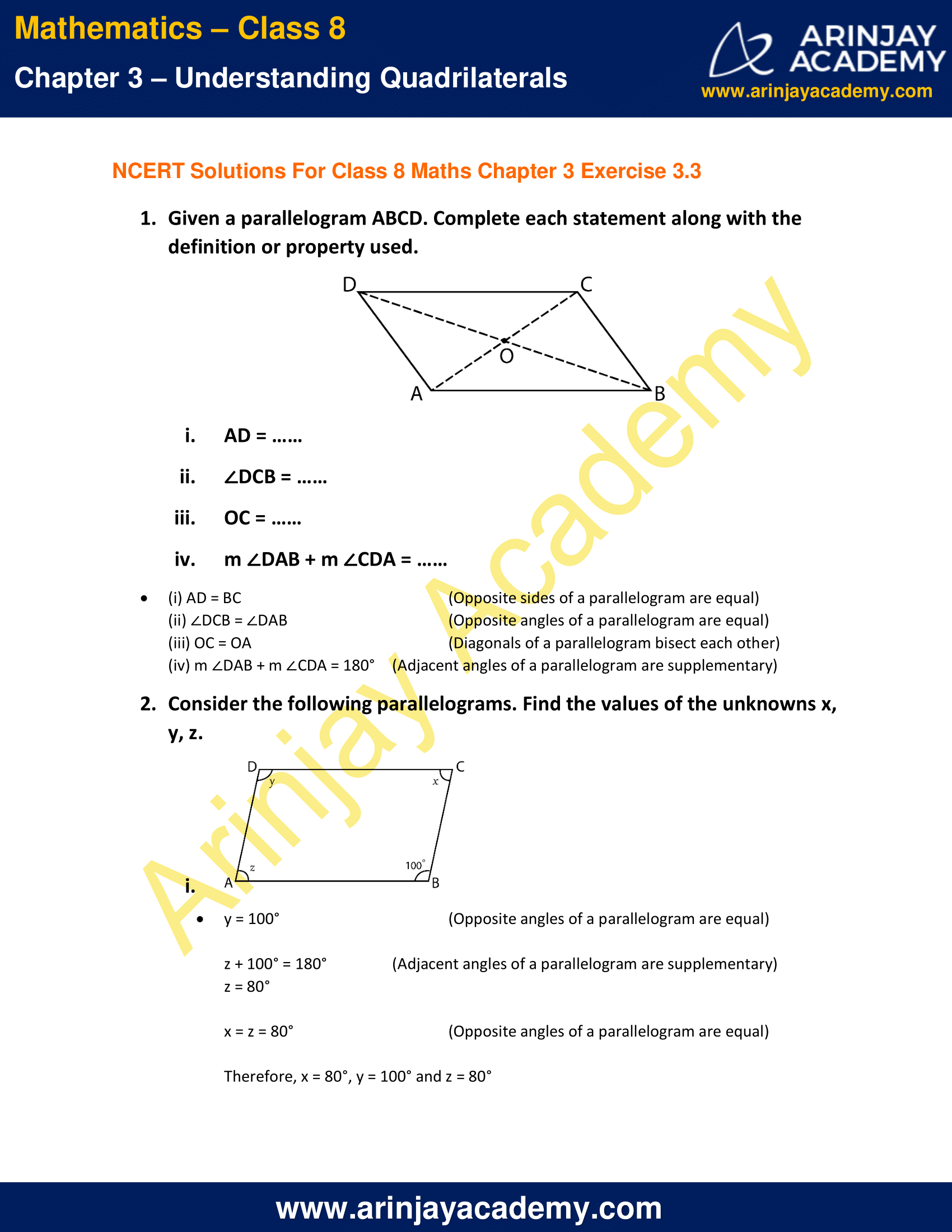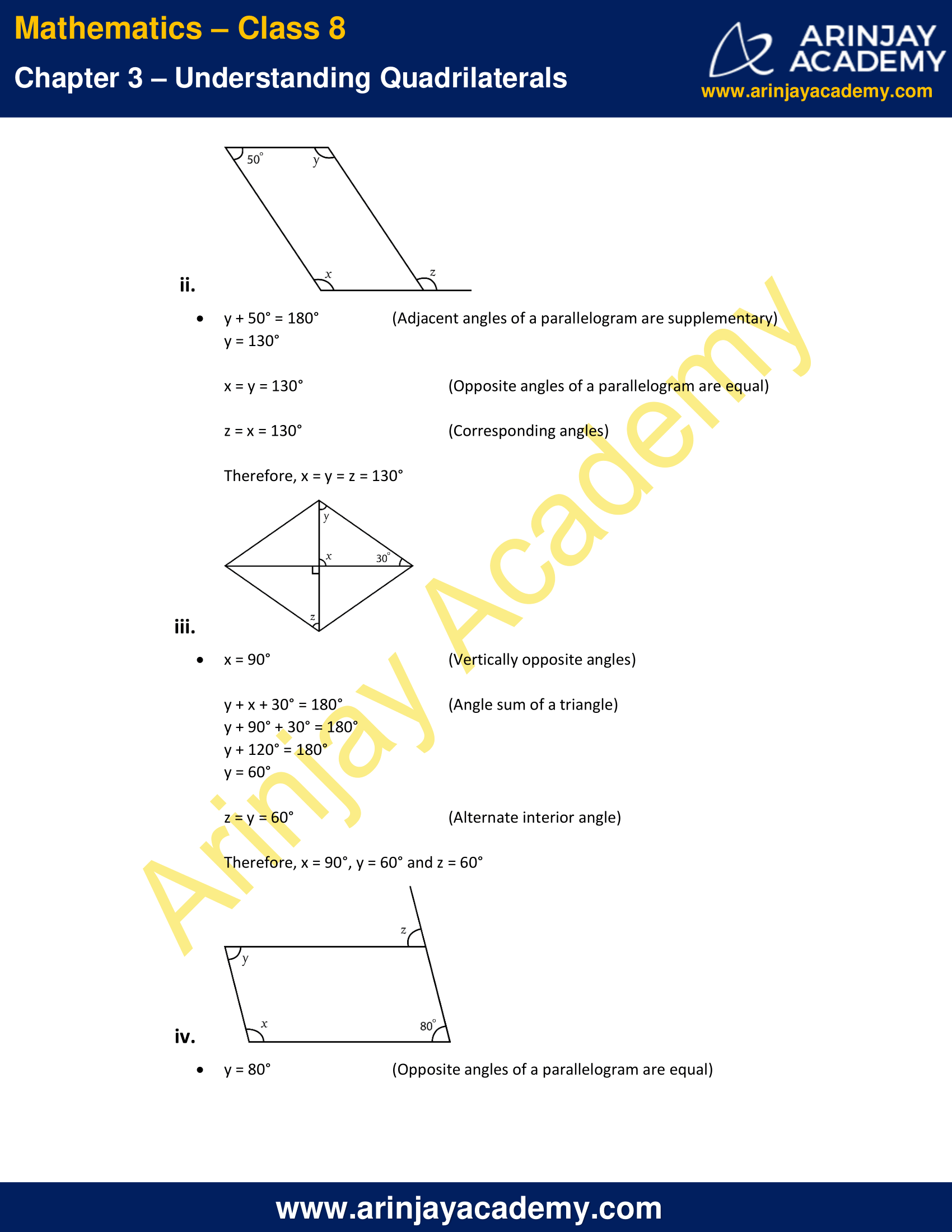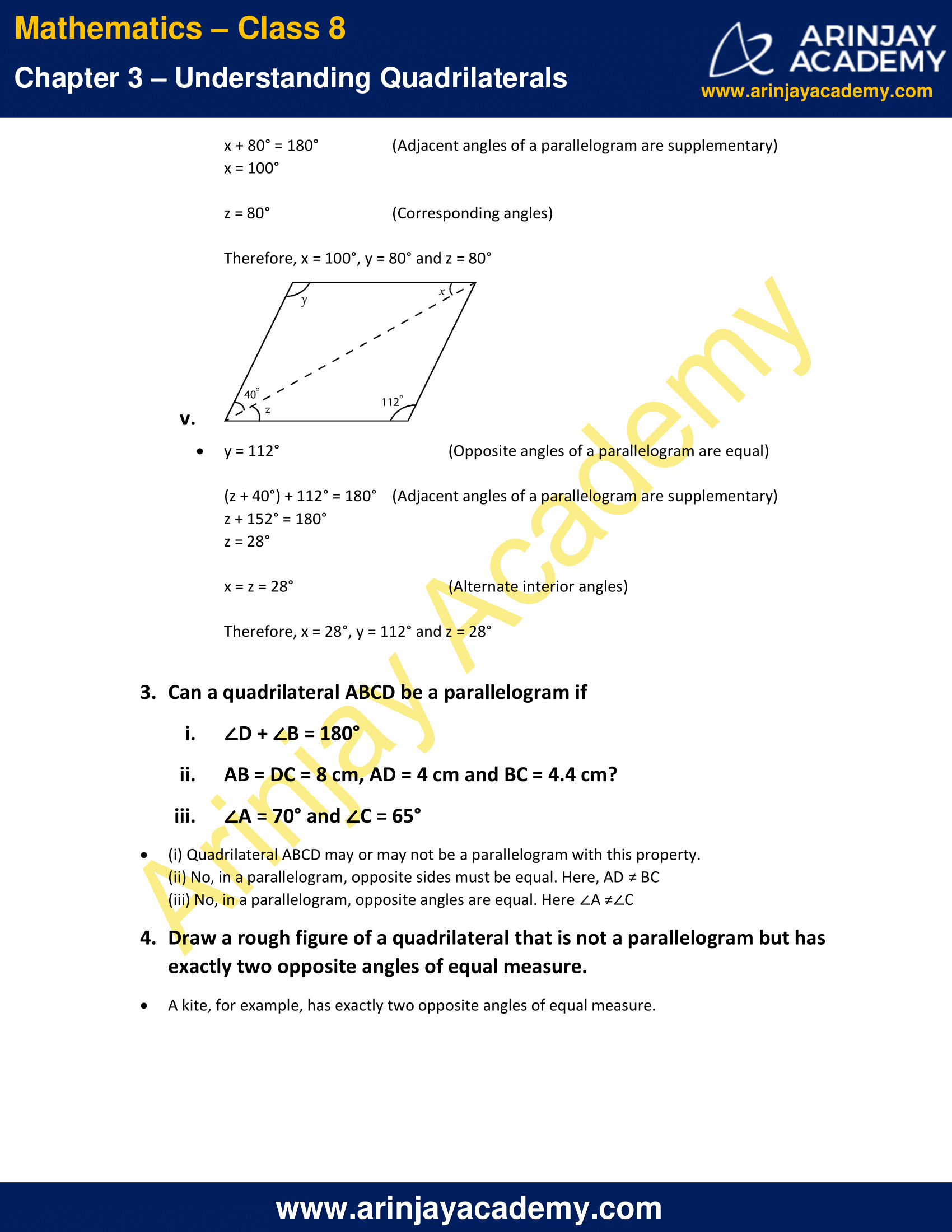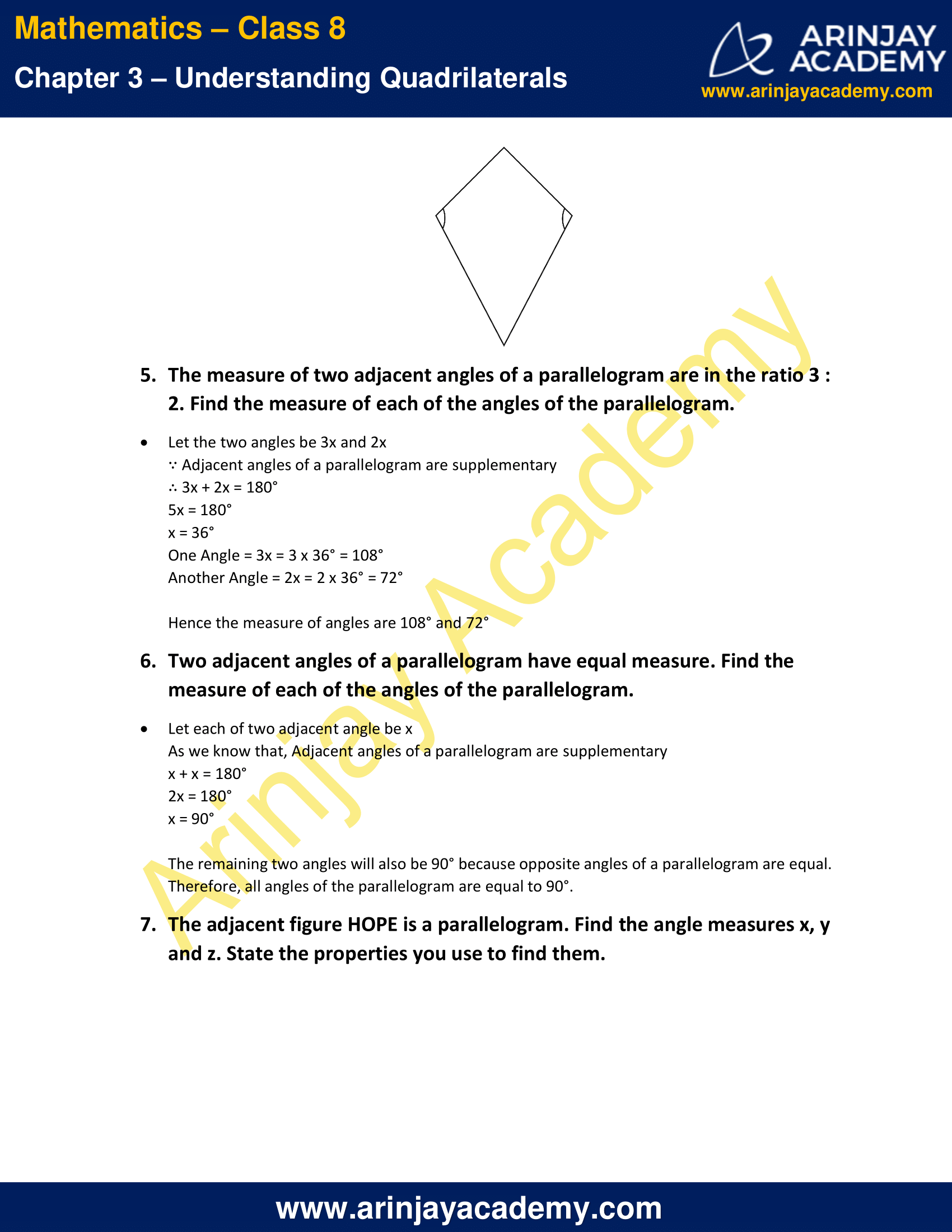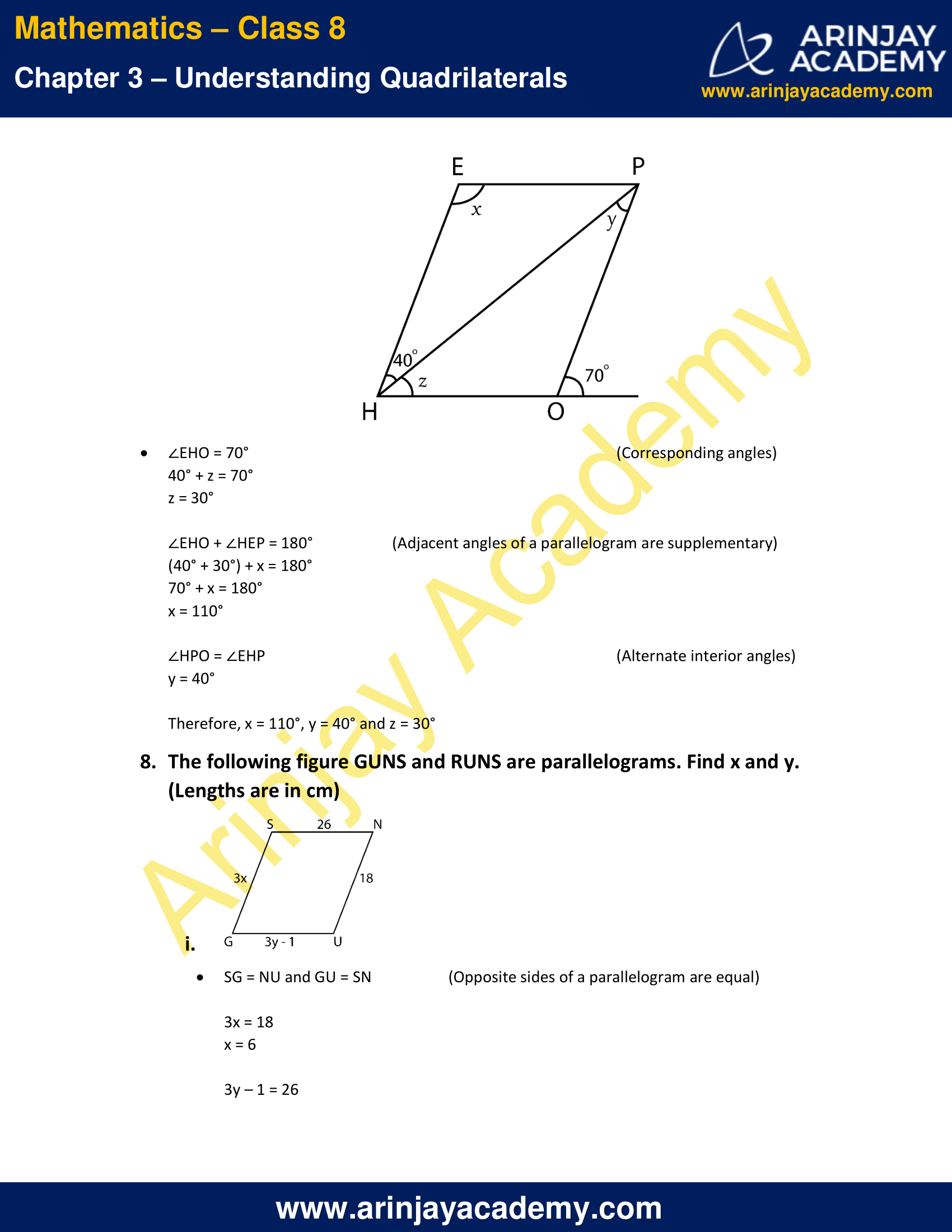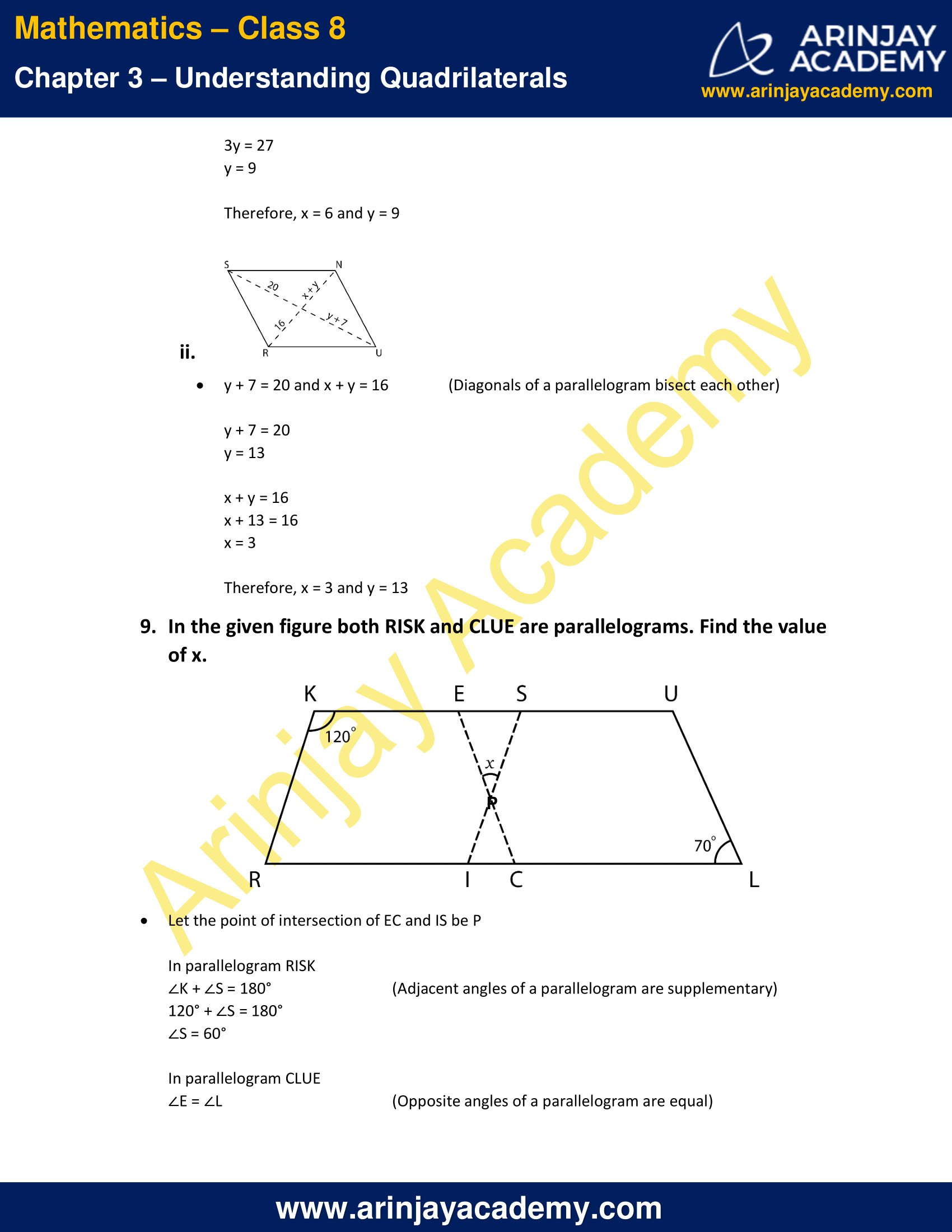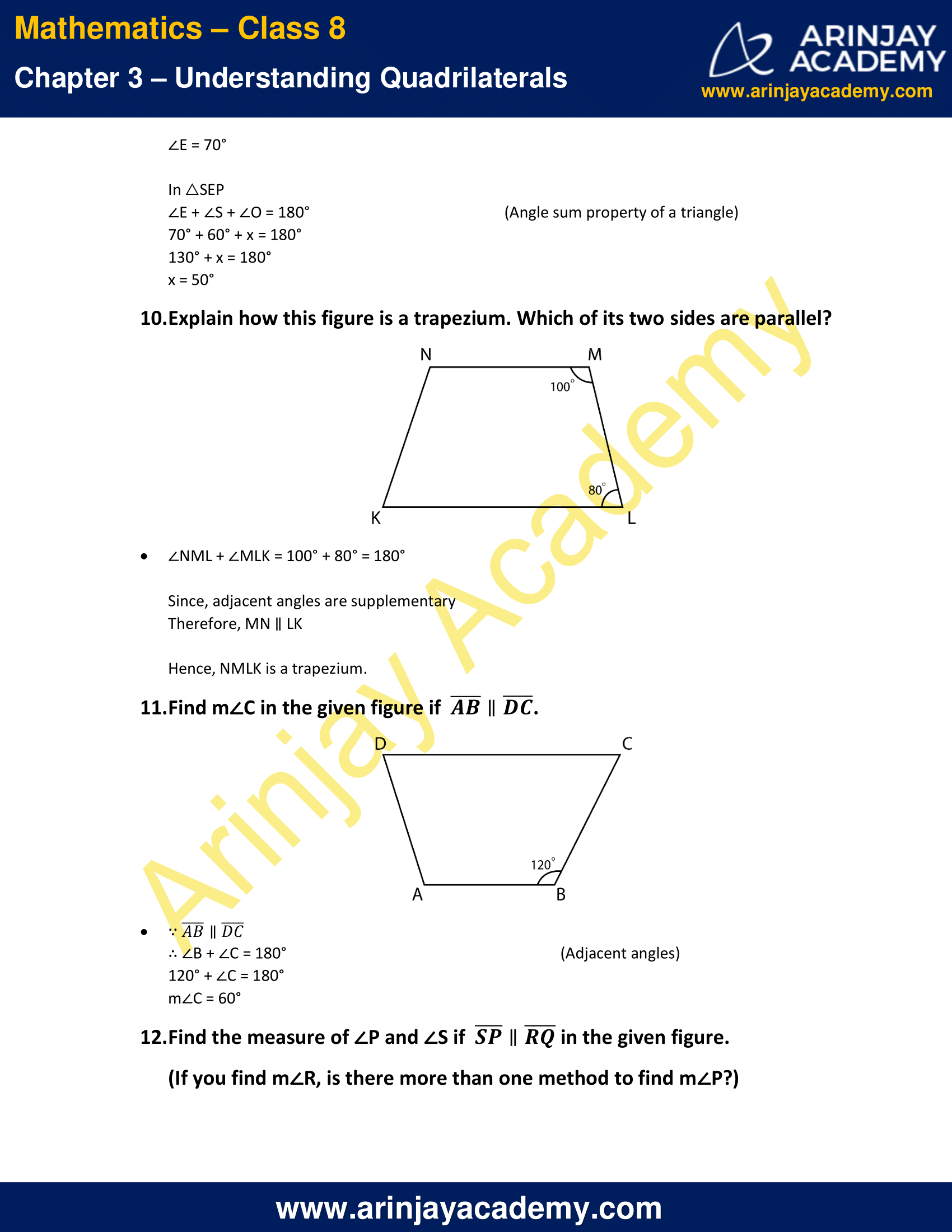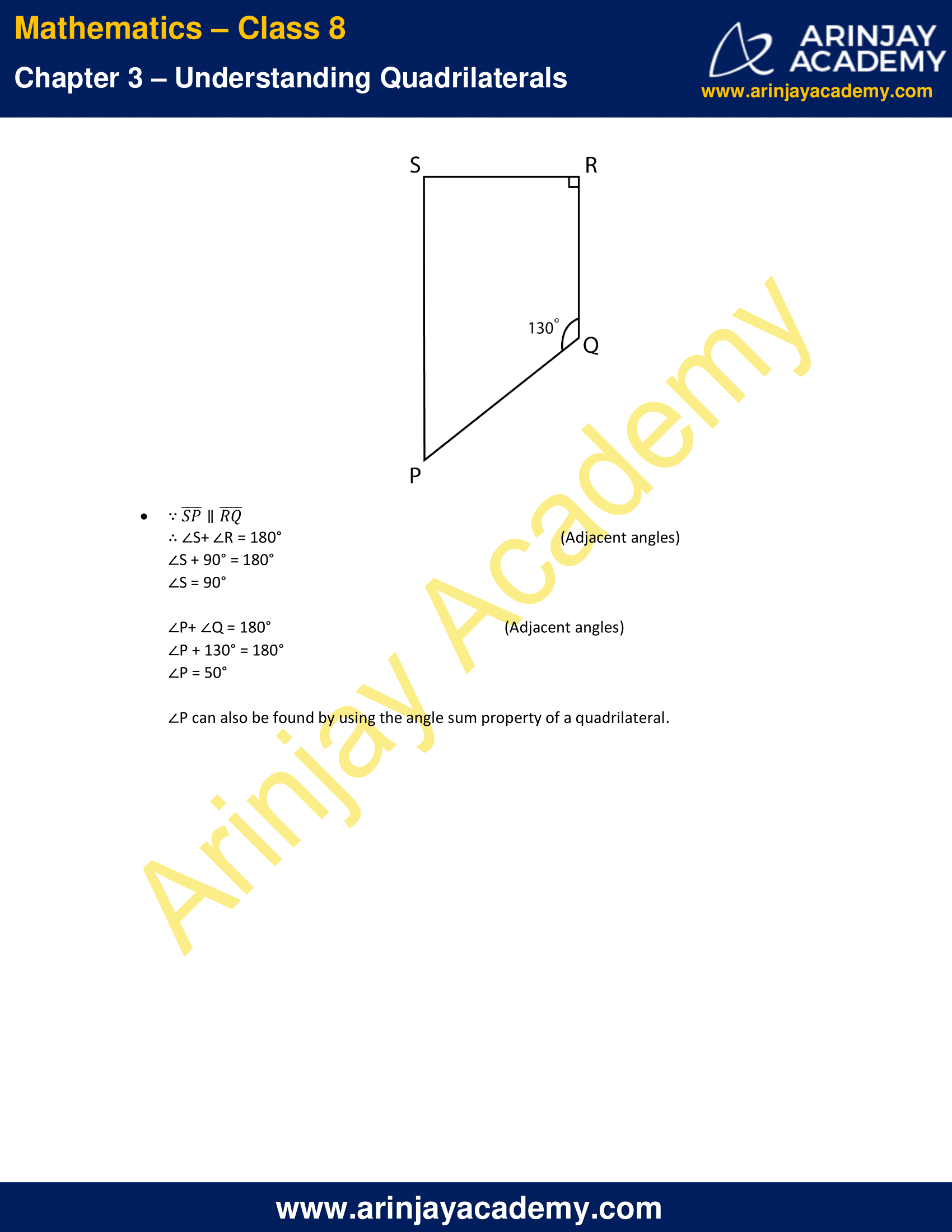NCERT Solutions for Class 8 Maths Chapter 3 Exercise 3.3

1. Given a parallelogram ABCD. Complete each statement along with the definition or property used.ii. ∠DCB = ……
iii. OC = ……
iv. m ∠DAB + m ∠CDA = ……

Solution:

(i) AD = BC (Opposite sides of a parallelogram are equal)
(ii) ∠DCB = ∠DAB  (Opposite angles of a parallelogram are equal)
(iii) OC = OA  (Diagonals of a parallelogram bisect each other)
(iv) m ∠DAB + m ∠CDA = 180° (Adjacent angles of a parallelogram are supplementary)

2. Consider the following parallelograms. Find the values of the unknowns x, y, z.

i.Solution:

y = 100° (Opposite angles of a parallelogram are equal)

z + 100° = 180° (Adjacent angles of a parallelogram are supplementary)
z = 80°

x = z = 80° (Opposite angles of a parallelogram are equal)

Therefore, x = 80°, y = 100° and z = 80°

ii.Solution:

∴Adjacent angles of a parallelogram are supplementary
y + 50° = 180°
y = 130°

x = y = 130°   (Opposite angles of a parallelogram are equal)

z = x = 130°   (Corresponding angles)

Therefore, x = y = z = 130°

iii.Solution:

x = 90° (Vertically opposite angles)

By Using Angle sum property of a triangle
y + x + 30° = 180°
y + 90° + 30° = 180°
y + 120° = 180°
y = 60°

z = y = 60° (Alternate interior angle)

Therefore, x = 90°, y = 60° and z = 60°

iv.Solution:

y = 80° (Opposite angles of a parallelogram are equal)

∴Adjacent angles of a parallelogram are supplementary
x + 80° = 180°
x = 100°

z = 80°  (Corresponding angles)

Therefore, x = 100°, y = 80° and z = 80°

v.Solution:

y = 112° (Opposite angles of a parallelogram are equal)

∴Adjacent angles of a parallelogram are supplementary
(z + 40°) + 112° = 180°
z + 152° = 180°
z = 28°

x = z = 28°   (Alternate interior angles)

Therefore, x = 28°, y = 112° and z = 28°

3. Can a quadrilateral ABCD be a parallelogram if

i. ∠D + ∠B = 180°
ii. AB = DC = 8 cm, AD = 4 cm and BC = 4.4 cm?
iii. ∠A = 70° and ∠C = 65°

Solution:

(i) Quadrilateral ABCD may or may not be a parallelogram with this property.
(ii) No, in a parallelogram, opposite sides must be equal. Here, AD ≠ BC
(iii) No, in a parallelogram, opposite angles are equal. Here ∠A ≠∠C

4. Draw a rough figure of a quadrilateral that is not a parallelogram but has exactly two opposite angles of equal measure.

Solution:

A kite, for example, has exactly two opposite angles of equal measure.5. The measure of two adjacent angles of a parallelogram are in the ratio 3 : 2. Find the measure of each of the angles of the parallelogram.

Solution:

Let the two angles be 3x and 2x
∵ Adjacent angles of a parallelogram are supplementary
∴ 3x + 2x = 180°
5x = 180°
x = 36°
One Angle = 3x = 3 x 36° = 108°
Another Angle = 2x = 2 x 36° = 72°
Hence, the measure of angles are 108° and 72°

6. Two adjacent angles of a parallelogram have equal measure. Find the measure of each of the angles of the parallelogram.

Solution:

Let each of two adjacent angle be x
As we know that, Adjacent angles of a parallelogram are supplementary
x + x = 180°
2x = 180°
x = 90°
The remaining two angles will also be 90° because opposite angles of a parallelogram are equal.
Therefore, all angles of the parallelogram are equal to 90°.

7. The adjacent figure HOPE is a parallelogram. Find the angle measures x, y and z. State the properties you use to find them.Solution:

∠EHO = 70° (Corresponding angles)
40° + z = 70°
z = 30°

∴Adjacent angles of a parallelogram are supplementary
∠EHO + ∠HEP = 180°
(40° + 30°) + x = 180°
70° + x = 180°
x = 110°

∠HPO = ∠EHP (Alternate interior angles)
y = 40°

Therefore, x = 110°, y = 40° and z = 30°

8. The following figure GUNS and RUNS are parallelograms. Find x and y. (Lengths are in cm)

i.Solution:

SG = NU and GU = SN (Opposite sides of a parallelogram are equal)
3x = 18
x = 6

3y – 1 = 26
3y = 27
y = 9

Therefore, x = 6 and y = 9

ii.Solution:

y + 7 = 20 and x + y = 16 (Diagonals of a parallelogram bisect each other)
y + 7 = 20
y = 13

x + y = 16
x + 13 = 16
x = 3

Therefore, x = 3 and y = 13

9. In the given figure both RISK and CLUE are parallelograms. Find the value of x.Solution:

Let the point of intersection of EC and IS be P

In parallelogram RISK
∴Adjacent angles of a parallelogram are supplementary
∠K + ∠S = 180°
120° + ∠S = 180°
∠S = 60°

In parallelogram CLUE
∠E = ∠L (Opposite angles of a parallelogram are equal)
∠E = 70°

By using Angle sum property of a triangle, In △SEP
∠E + ∠S + ∠O = 180°
70° + 60° + x = 180°
130° + x = 180°
x = 50°

10. Explain how this figure is a trapezium. Which of its two sides are parallel?Solution:

∠NML + ∠MLK = 100° + 80° = 180°

Therefore, MN ∥ LK

Hence, NMLK is a trapezium.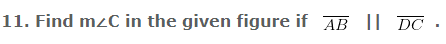Solution: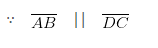∴ ∠B + ∠C = 180° (Adjacent angles)
120° + ∠C = 180°
m∠C = 60°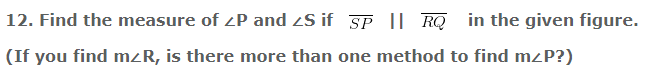Solution: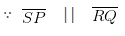∴ ∠S+ ∠R = 180°  (Adjacent angles)
∠S + 90° = 180°
∠S = 90°

∠P+ ∠Q = 180°  (Adjacent angles)
∠P + 130° = 180°
∠P = 50°

∠P can also be found by using the angle sum property of a quadrilateral.

The next Exercise for NCERT Solutions for Class 8 Maths Chapter 3 Exercise 3.4 – Understanding Quadrilaterals can be accessed by clicking here